MORE IN Structural Analysis 1
SPPU Civil Engineering (Semester 4)
Structural Analysis 1
May 2015
Total marks: --
Total time: --
INSTRUCTIONS
(1) Assume appropriate data and state your reasons
(2) Marks are given to the right of every question
(3) Draw neat diagrams wherever necessary

Answer any one question from Q1 and Q2
1 (a) A 4 m simply supported beam subjected to clockwise moment 20 kNm at mid span, determine maximum slope and deflection in term of EI.
6 M
1 (b) Determine moment at B for the continuous beam loaded an supported as shown in the Fig. 1(b) by Clapeyron's theorem.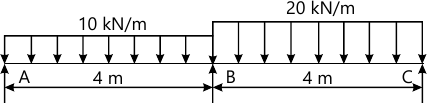6 M

2 (a) A propped cantilever of span 3 m loaded with uniformly distributed load 10 kN/m on entire span, determine the prop reaction.
6 M
2 (b) Determine the fixed end moments for the fixed beam loaded and supported as shown in Fig. 2(b).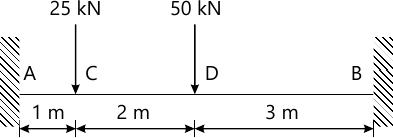6 M

Answer any one question from Q3 and Q4
3 (a) Find the horizontal deflections of joint C of the truss shown in Fig. 3(a). The area of inclined member is 2000 mm2 while the area of horizontal member is 1600 mm2. Take E=200 kN/mm2.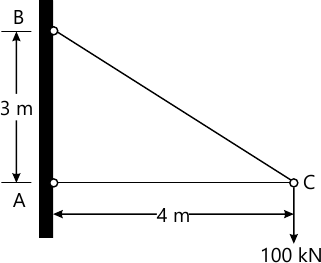6 M
3 (b) A simply supported beam is loaded and supported as shown in Fig. 3(b). Determine support reaction at A. Shear and moment at C by drawing Influence line diagram.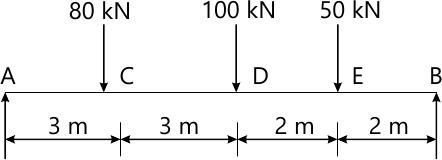6 M

4 (a) Find force in members of the truss as shown in Fig. 4(a). Cross-sectional area and material of all members is same.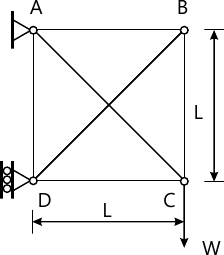6 M
4 (b) Draw the influence line diagram for the members U2U3, L2L3 and U2L2 of a trus as shown in Fig. 4(b)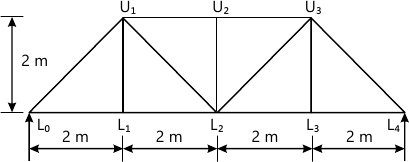6 M

Answer any one question from Q5 and Q6
5 (a) A three hinge parabolic arch has a span of 24 m and a central rise of 4 m. It carries a concentrated load of 50 kN at 18m from left support. Determine thrust and radial shear at a section 6m from left support.
7 M
5 (b) Derive the expression for horizontal thrust when a uniformly distributed load w is acting on entire span of two hinged semicircular arch.
6 M

6 (a) A three hinged circular arch has a span of 40 m and a central rise of 8 m. It carries a uniformly distributed load 20 kN/m over the left-half of span. Find the reaction at the supports and shear at a section 10m front left support.
7 M
6 (b) A two hinged parabolic arch of span 30 m and central rise 4m is subjected to a point load of 30 kN at the center of the arch. Find the horizontal thrust and moment at 8 m from left hand support.
6 M

Answer any one question from Q7 and Q8
7 (a) State and explain lower bound, upper bound and uniqueness theorem.
5 M
7 (b) A beam fixed at both ends is subjected to central point load W. The beam is of uniform plastic moment Mp. Determine the magnitude of collapse load.
8 M

8 (a) Find the shape factor for circular cross-section of diameter f.
5 M
8 (b) Determine collapse load in propped cantilever of span L subjected to central concentrated load W.
8 M

More question papers from Structural Analysis 1